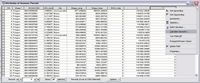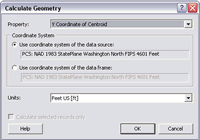ArcWatch: Your e-Magazine for GIS News, Views, and Insights

#### January 2008

New Tool Eliminates Need for Custom Code

## Calculate Geometry in Table FieldsAfter creating a field to hold the calculated values, right-click on the heading and choose Calculate Geometry.In the Calculate Geometry dialog box, choose the property to calculate, coordinate system, and units.

At ArcGIS Desktop 9.2, a new tool in ArcMap lets you perform calculations on the feature geometry in the attribute tables of feature layers. The Calculate Geometry tool calculates lengths; areas; and x,y coordinates. In previous releases, these operations were carried out by writing custom Visual Basic for Applications (VBA) code. Now these fields can be generated through a simple menu choice.

The geometry that can be calculated depends on the feature used. Polygon features have the most options: area, perimeter, x-coordinate of centroid, and y-coordinate of centroid. Point features have the fewest choices: x-coordinate of point and y-coordinate of point. The x-coordinate of centroid and y-coordinate of centroid can be calculated for line and annotation features. The length of line features can also be calculated.

These calculations take place in projected, rather than spherical or geodesic, space. For data sources in a geographic coordinate system, such as World Geodetic System (WGS) 1984 (i.e., unprojected), the projected coordinate system of the data frame can be used for calculations.

1. To use this tool, add a field in the attribute table for the layer to hold the calculated data first. For the most precise results, set the field type to double.
2. Access Calculate Geometry by right-clicking the heading for the field just created and choose Calculate Geometry from the context menu.
3. In the Calculate Geometry dialog box, choose what you wish to calculate from the drop-down list next to Property. Pick a coordinate system, either the one used by the data source or by the data frame, and select the units to be used.
4. Click OK. The field will be populated with new calculated values.

If the geometry for only a subset of the attribute table is required, create a selection of that subset before using Calculate Geometry and the tool will calculate values only for selected records.